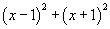# expression

Expression

Any mathematical calculation or formula combining numbers and/or variables using sums, differences, products, quotients (including fractions), exponents, roots, logarithms, trig functions, parentheses, brackets, functions, or other mathematical operations. Expressions may not contain the equal sign (=) or any type of inequality.

Examples: